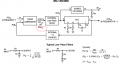# Frequency multiplier which allow adjust the duty cycle

#### nataraj marble

Joined Aug 30, 2021
1
I am currently working on frequency multipliers now. Is there a frequency multiplier topology where the input and output can be on the same duty cycle? For example, if input frequency have 20% of duty cycle then the output frequency signal should be 20% of duty cycle. Generally using PLL method, if frequency is multiplied with even numbers then you will give 50% of duty cycle. But ı want to get same duty cycle with input. Thank you for your help in advance.

#### BobTPH

Joined Jun 5, 2013
6,305
What frequency range?

Voltage range?

Square wave only or arbitrary?

Does output voltage also follow input?

#### ronsimpson

Joined Oct 7, 2019
2,620
I have used the CD4046 or MC14046 PLL in "edge lock" mode. In a 10X frequency multiplier it will lock the rising edge of the reference frequency to the tenth rising edge of the output frequency. That gives you the 10x frequency using a IC that does not look at the duty cycle or the falling edge.

Many PLL get upset when the duty cycle changes. That is why I think you need a edge lock loop.

We need to make a "duty cycle lock loop" if there is such a thing. Use a simple RC to find the duty cycle of the input frequency. Example; 50% duty cycle gives you half the supply voltage. Also make the same circuit to monitor the duty cycle of the output frequency. Compare the two voltages. This tells you how much to adjust the oscillator to make the duty cycle to match.

I cannot remember now but I had a way to set the duty cycle of the 4046. I need to think about that.